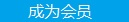您可以捐助，支持我们的公益事业。 1元 10元 50元 认证码：必填求知 文章 文库 Lib 视频 Code iProcess 课程 认证 咨询 工具 火云堂 讲座吧 成长之路要资料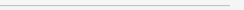订阅捐助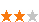292 次浏览     评价： 好 中 差
2019-1-2

 编辑推荐: 本文来自于ibm，文章介绍了卷积网络的工作原理以及如何使用 Python 实现一个对手写数字进行分类的简单网络。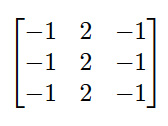def basic_conv(image, out, in_width, in_height, out_width, out_height, filter, filter_dim, stride): result_element = 0 for res_y in range(out_height): for res_x in range(out_width): for filter_y in range(filter_dim): for filter_x in range(filter_dim): image_y = res_y + filter_y image_x = res_x + filter_x result_element += (filter[filter_y][filter_x] * image[image_y][image_x]) out[res_y][res_x] = result_element result_element = 0 res_x += (stride - 1) res_y += (stride - 1) return out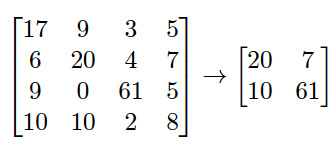def max_pool(input, out, in_width, in_height, out_width, out_height, kernel_dim, stride): max = 0 for res_y in range(out_height): for res_x in range(out_width): for kernel_y in range(kernel_dim): for kernel_x in range(kernel_dim): in_y = (res_y * stride) + kernel_y in_x = (res_x * stride) + kernel_x if input[in_y][in_x] > max: max = input[in_y][in_x] out[res_y][res_x] = max max = 0 return out

 def make_conv_layer(self, input, in_channels, out_channels): layer_weights = self.init_conv_weights(in_channels, out_channels) layer_bias = self.make_bias_term(out_channels) layer_activations = tf.nn.conv2d(input, layer_weights, strides = self.conv_strides, padding = self.conv_padding) + layer_bias return self.relu(layer_activations)

 # Layer 2: Max Pooling.28x28x6 --> 14x14x6 p2 = self.make_pool_layer(c1) # Layer 3. convolutional, ReLU nonlinearity, 14x14x6 --> 10x10x16 c3 = self.make_conv_layer(p2, 6, 16) # Layer 4.Max Pooling.10x10x16 --> 5x5x16 p4 = self.make_pool_layer(c3) # Flattening the features to be fed into a fully connected layer fc5 = self.flatten_input(p4) # Layer 5.Fully connected.400 --> 120 fc5 = self.make_fc_layer(fc5, 400, 120) # Layer 6.Fully connected.120 --> 84 fc6 = self.make_fc_layer(fc5, 120, 84) # Layer 7.Fully connected.84 --> 10.Output layer, so no ReLU. fc7 = self.make_fc_layer(fc6, 84, 10, True) return fc7

 x_train, y_train, x_valid, y_valid, x_test, y_test = split() x_train = pad(x_train) x_valid = pad(x_valid) x_test = pad(x_test) x_train_tensor = tf.placeholder(tf.float32, (None, 32, 32, 1)) y_train_tensor = tf.placeholder(tf.int32, (None)) y_train_one_hot = tf.one_hot(y_train_tensor, 10) !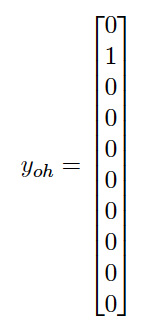net = lenet.LeNet5() logits = net.run_network(x_train_tensor) learn_rate = 0.001 cross_ent = tf.nn.softmax_cross_entropy_with_logits(logits = logits, labels = y_train_one_hot) loss = tf.reduce_mean(cross_ent) # We want to minimise the mean cross entropy optimisation = tf.train.AdamOptimizer(learning_rate = learn_rate) train_op = optimisation.minimize(loss) correct = tf.equal(tf.argmax(logits, 1), tf.argmax(y_train_one_hot, 1)) accuracy = tf.reduce_mean(tf.cast(correct, tf.float32))

 with tf.Session() as sess: sess.run(tf.global_variables_initializer()) example_count = len(x_train) for i in range(num_epochs): x_train, y_train = shuffle(x_train, y_train) for j in range(0, example_count, batch_size): batch_end = j + batch_size batch_x, batch_y = x_train[j : batch_end], y_train[j : batch_end] sess.run(train_op, feed_dict = {x_train_tensor: batch_x, y_train_tensor: batch_y}) accuracy_valid = eval(x_valid, y_valid) print("Accuracy: {}".format(accuracy_valid)) print() save.save(sess, "SavedModel/Saved")292 次浏览  评价: 好 中 差订阅捐助
 相关文章 我们该如何设计数据库 数据库设计经验谈 数据库设计过程 数据库编程总结
 相关文档 数据库性能调优技巧 数据库性能调整 数据库性能优化讲座 数据库系统性能调优系列
 相关课程 高性能数据库设计与优化 高级数据库架构师 数据仓库和数据挖掘技术 Hadoop原理、部署与性能调优每天2个文档/视频 扫描微信二维码订阅
 订阅技术月刊 获得每月300个技术资源